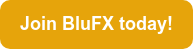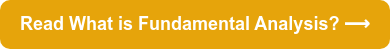Margin in forex is the amount of money that a trader needs to put forwards in order to open a trade. Margin can either be used or free - you can find out the difference between the two below.

Understanding margin a crucial part of trading - so it’s important you know the basics. Here’s our guide to margin, including: how to calculate margin in forex and the difference between used and free margin...

Interested in how BluFX margin works? Read more on our BluFX Margin Explained blog.# What is margin in forex?

As explained above, margin in forex is the amount of money that a trader needs to put forwards in order to open a trade.

Think of margin as a deposit that the trader needs to place down in order to keep a trade open.

This money is held by the broker. The total amount of money that the broker holds to keep the trader’s positions open is called used margin.

For example, in order to control a \$100,000 position, your broker will set aside \$1,000 from your account. So you can now control \$100,000 with \$1,000.

You can think of this in a similar way to a house deposit: you pay 10% up front to secure the whole house.

Margin is usually expressed as a percentage of the full amount of the position: for example, brokers will say they require 1% margin, 5% margin, and so on.

So - how does margin work at BluFX? Visit our blog, BluFX Margin Explained, to find out more.

### What is free margin in forex?

Used margin, as explained above, is the total amount of money that the broker holds to keep the trader’s positions open, like a deposit. So - what is free margin?

Free margin in forex (also known as ‘usable margin’) is the available money in a trader’s account to open new positions. In other words, money that is NOT tied up in margin for current open positions.

For example, if you had \$10,000 in your account and \$8,000 in used margin, the free margin would be \$2,000.

So, to calculate free margin: equity - used margin.

### How to calculate margin level in forex

After this, the money that a trader has left available to open other positions is called available equity - and this can be expressed as a percentage called margin level.

Margin level provides a measure of how well the trading account is funded by dividing equity by the used margin and then multiplying this figure by 100.

Margin level needs to be above 100% in order to keep opening trades. If the level drops to 100%, this means that all available margin is in use and the trader can no longer open new trades. If it drops below 100%, this is known as margin call - see more about this below.

For example, \$10,000 / \$8,000 x 100 = 125%.

So, to calculate margin level in forex: equity / used margin x 100.

### What is margin call?

Margin call happens when your equity falls below your used margin (for example, if your equity is \$8,000 but your used margin is \$12,000). Margin call means that the margin level (as discussed above) has fallen below 100%.

Essentially, this means that the amount of money in your account cannot cover any possible loss. If this happens, the broker will close some or all open positions - so margin call is something traders should try to avoid at all costs!

How can a country’s political climate affect its currency? Find out in Fundamental Analysis 101...Tags: Margin